# Rectangle and circle

The rectangle ABCD has side lengths a = 40 mm and b = 30 mm and is circumscribed by a circle k. Calculate approximately how many cm is circle long.

Correct result:

x =  157.08 mm

#### Solution: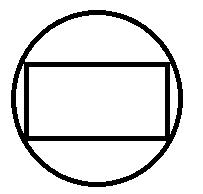We would be pleased if you find an error in the word problem, spelling mistakes, or inaccuracies and send it to us. Thank you!Tips to related online calculators
Do you want to convert length units?
Pythagorean theorem is the base for the right triangle calculator.

#### You need to know the following knowledge to solve this word math problem:

We encourage you to watch this tutorial video on this math problem:

## Next similar math problems:

• TentCalculate how many liters of air will fit in the tent that has a shield in the shape of an isosceles right triangle with legs r = 3 m long the height = 1.5 m and a side length d = 5 m.
• Central park in city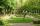The city park has the shape of a rectangle of 180 meters in length and 120 meters in width. People make their walk through the center of the park from one corner to the second. Calculate how many meters this way is shorter than they walked along the path
• Rhombus and inscribed circleIt is given a rhombus with side a = 6 cm and the radius of the inscribed circle r = 2 cm. Calculate the length of its two diagonals.
• Rectangle - parallelogram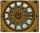It is given a rectangle that is circumscribed by a circle with a radius of 5 cm. The short side of the rectangle measures 6 cm. Calculate the perimeter of a parallelogram ABCD whose vertices are the midpoints of the sides of the rectangle.
• GardenArea of a square garden is 6/4 of triangle garden with sides 56 m, 35 m, and 35 m. How many meters of fencing need to fence a square garden?
• Similarity coefficientThe ratio of similarity of two equilateral triangles is 3.5 (ie 7:2). The length of the side of smaller triangle is 2.4 cm. Calculate the perimeter and area of ​​the larger triangle.
• Rhombus ABCDRhombus ABCD, |AC| = 90 cm, |BD| = 49 cm. Calculate the perimeter of the rhombus ABCD.
• DiagonalCalculate the length of the diagonal of the rectangle ABCD with sides a = 8 cm, b = 7 cm.
• Rectangle - desc circle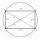Length of the sides of the rectangle are at a ratio 1: 3 . Radius of the circle circumscribed to rectangle is 10 cm. Calculate the rectangle's perimeter.
• CanopyMr Peter has metal roof cone shape with a height of 127 cm and radius 130 cm over well. He needs paint the roof with anticorrosion. How many kg of color must he buy if the manufacturer specifies the consumption of 1 kg to 3.3 m2?
• IS trapezoidCalculate the length of diagonal u and height v of isosceles trapezoid ABCD, whose bases have lengths a = |AB| = 37 cm, c = |CD| = 29 cm and legs b = d = |BC| = |AD| = 28 cm.
• RectangleThe rectangle is 21 cm long and 38 cm wide. Determine the radius of the circle circumscribing rectangle.
• Right trapezoidThe right trapezoid has bases 3.2 cm and 62 mm long. The shorter leg has a length 0.25 dm. Calculate the lengths of the diagonals and the second leg.
• Square circles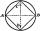Calculate the length of the described and inscribed circle to the square ABCD with a side of 5cm.
• Area 4gonCalculate area of 4-gon, two and the two sides are equal and parallel with lengths 11, 5, 11 and 5. Inner angles are 45°, 135°,45°, 135°.
• Recursion squaresIn the square ABCD is inscribed a square so that its vertices lie at the centers of the sides of the square ABCD.The procedure of inscribing square is repeated this way. Side length of square ABCD is a = 22 cm. Calculate: a) the sum of perimeters of all s
• TV diagonalDiagonal TV is 0.56 m long, how big the television sreen is if the aspect ratio is 16:9?